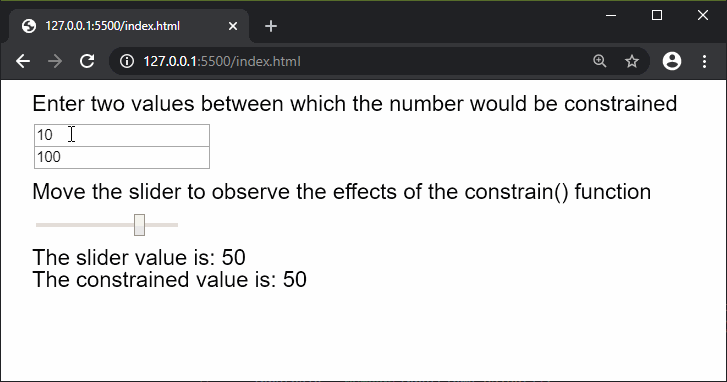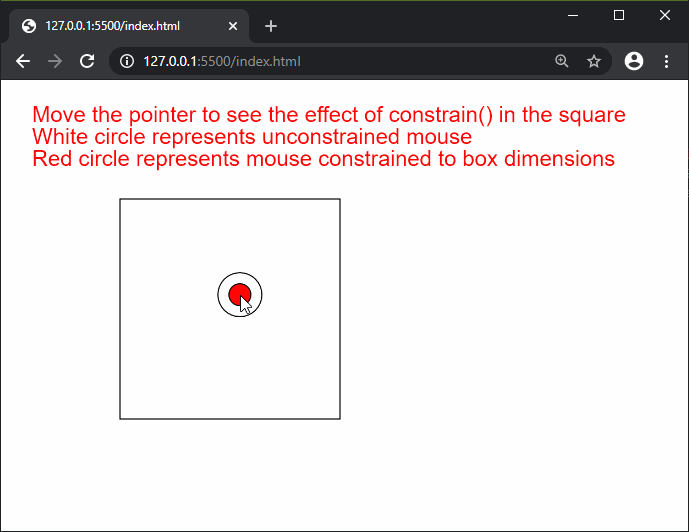Open in App
Not now

# p5.js | constrain() Function

• Last Updated : 25 Mar, 2020

The constrain() function in p5.js is used to constrain a number between a given minimum and maximum limit.

Syntax:

`constrain( n, low, high )`

Parameters: This function accept three parameters as mentioned above and described below:

• n: It is a number which denotes the value that has to be constrained.
• low: It is a number which denotes the minimum limit to which the number is constrained.
• high: It is a number which denotes the maximum limit to which the number is constrained.

Return Value: It returns a number with the constrained value.

The example below illustrates the constrain() function in p5.js:

Example 1:

 `function` `setup() { ` `  ``createCanvas(650, 200); ` `  ``textSize(20); ` `  `  `  ``inputElemA = createInput(10); ` `  ``inputElemA.position(30, 40); ` `  `  `  ``inputElemB = createInput(100); ` `  ``inputElemB.position(30, 60); ` `  `  `  ``sliderElem = createSlider(-100, 100, 50, 1); ` `  ``sliderElem.position(30, 120); ` `} ` `  `  `function` `draw() { ` `  ``clear(); ` `  ``text(``"Enter two values between which the "` `    ``+ ``"number would be constrained"``, 20, 20); ` `  ``text(``"Move the slider to observe the effects"` `    ``+ ``" of the constrain() function"``, 20, 100); ` `  `  `  ``// Convert the string value to a number value ` `  ``inputValA = Number(inputElemA.value()); ` `  ``inputValB = Number(inputElemB.value()); ` `  ``sliderVal = sliderElem.value(); ` `  `  `  ``text(``"The slider value is: "` `+ sliderVal, 20, 160); ` `  `  `  ``// Display the constrained value ` `  ``text(``"The constrained value is: "` `        ``+ constrain(sliderVal, inputValA, ` `        ``inputValB), 20, 180); ` `} `

Output:Example 2:

 `function` `setup() { ` `  ``createCanvas(600, 350); ` `  ``textSize(20); ` `  `  `} ` `  `  `function` `draw() { ` `  ``clear(); ` `  ``text(``"Move the pointer to see the effect "` `        ``+ ``"of constrain() in the square"``, 20, 30); ` `  ``text(``"White circle represents unconstrained"` `        ``+ ``" mouse"``, 20, 50); ` `  ``text(``"Red circle represents mouse constrained"` `        ``+ ``" to box dimensions"``, 20, 70); ` `  `  `  ``noFill(); ` `  ``square(100, 100, 200); ` `  `  `  ``circle(mouseX, mouseY, 40); ` `  `  `  ``// Constrain the mouse x and y position ` `  ``constrainedMouseX = constrain(mouseX, 100, 300); ` `  ``constrainedMouseY = constrain(mouseY, 100, 300); ` `  `  `  ``fill(``'red'``); ` `  ``circle(constrainedMouseX, constrainedMouseY, 20); ` `} `

Output:Online editor: https://editor.p5js.org/

My Personal Notes arrow_drop_up
Related Articles